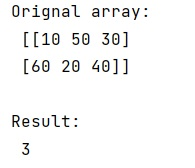# Get the position of the largest value in a multi-dimensional NumPy array

Learn, how to get the position of the largest value in a multi-dimensional NumPy array in Python?
Submitted by Pranit Sharma, on January 11, 2023

NumPy is an abbreviated form of Numerical Python. It is used for different types of scientific operations in python. Numpy is a vast library in python which is used for almost every kind of scientific or mathematical operation. It is itself an array which is a collection of various methods and functions for processing the arrays.

## Getting the position of the largest value in a multi-dimensional

Suppose that we are given a multi-dimensional array with some integer values and we need to find the position of the largest value in this array.

Whenever we need to find the position of the max element, we always use the argmax() method. Although this method is generally used with one-dimensional arrays, the method would work for multidimensional arrays as well.

Let us understand with the help of an example,

## Python code to get the position of the largest value in a multi-dimensional NumPy array

```# Import numpy
import numpy as np

# Creating numpy array
arr = np.array([[10,50,30],[60,20,40]])

# Display original array
print("Orignal array:\n",arr,"\n")

# Finding the position of max element
res = arr.argmax()

# Display result
print("Result:\n",res,"\n")
```

Output:What's New (MCQs)

Top Interview Coding Problems/Challenges!

IncludeHelp's Blogs

Languages: » C » C++ » C++ STL » Java » Data Structure » C#.Net » Android » Kotlin » SQL
Web Technologies: » PHP » Python » JavaScript » CSS » Ajax » Node.js » Web programming/HTML
Solved programs: » C » C++ » DS » Java » C#
Aptitude que. & ans.: » C » C++ » Java » DBMS
Interview que. & ans.: » C » Embedded C » Java » SEO » HR
CS Subjects: » CS Basics » O.S. » Networks » DBMS » Embedded Systems » Cloud Computing
» Machine learning » CS Organizations » Linux » DOS
More: » Articles » Puzzles » News/Updates